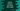# How to compare one string with integer value in python## How to compare one string with integer value in python:

Both string and integer are of different types. We can compare two strings or two integers. Python uses lexicographic ordering for strings and numeric ordering for integers.

But how can we compare one string with an integer value in python ? For that, we need to convert the string value to integer using int() constructor. Or we can convert it to a float using float().

### Exception:

If we don’t convert and try to compare one string with an integer, it will throw one TypeError :

``````given_str = '5'
given_int = 10

print(given_int > given_str)``````

It will print:

``````Traceback (most recent call last):
File "example.py", line 4, in <module>
print(given_int > given_str)
TypeError: '>' not supported between instances of 'int' and 'str'``````

### Python program to compare string and integer:

Let me change the above program with string to integer conversion:

``````given_str = '5'
given_int = 10

print(given_int > int(given_str))``````

It will print:

``True``

### Exception on invalid values:

For invalid inputs, it will throw one error. For example,

``````given_str = '5xx'
given_int = 10

print(given_int > int(given_str))``````

Here, given_str is not a valid integer value. So, it will throw one error:

``````Traceback (most recent call last):
File "example.py", line 4, in <module>
print(given_int > int(given_str))
ValueError: invalid literal for int() with base 10: '5xx'``````